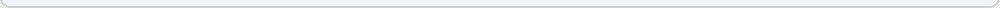# Quarterly Compounded Dividend Calculator

Dividend Reinvestment is where you reinvest your dividends in the same stock that issues the dividend originally, then the next time the dividend is issued you have more shares, so your dividend is higher, and you reinvest more, thus gaining more shares. This is called compounding, and can make you very wealthy in the long term. The more frequent the distributions, the more frequent the compounding, the more money you will make. This calculator is a quarterly compounded dividend calculator it is not as "slow" as our Annually Compounded Dividend Calculator nor as "fast" as our Monthly Compounded Dividend Calculator, however the vast majority of equities that issue dividends do so quarterly, as such this one is usually the most accurate for calculating the returns of most stocks.

 Starting Yield: % (the current annual dividend yield, such as 4.5) Dividend Growth Rate: % (the historical average increase in the dividend as a percentage, you may set this to 0 to disregard it) Number of Shares Held: (the number of shares you own in the stock) Cost per Share: \$ (your purchase price for the shares) Years to hold: (the number of years for which you want to calculate results)